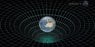# General theory of relativity&Planet orbits

• B
• Sai Siddhartha k
In summary, the general theory of relativity is generally accurate and applicable, and planetary orbits are elliptical, with small perturbations.

#### Sai Siddhartha k

Hi all,
Only one is true at a time "general theory of relativity or Planetary orbits are elliptical...please clarify me I will thank full to you..

Assuming that I am correctly comprehending and/or inferring what you are asking,

The statements:
"[The] general theory of relativity [is generally accurate and applicable]"
AND
"Planetary orbits are elliptical."

are both generally true and accurate (in standard usage and understanding) and are in no way mutually exclusive or contradictory.

... or perhaps I misunderstood what is being asked.

diogenesNY

•Klystron
the force of gravity in fact arises from the curvature of space and time. Einstein proposed that objects such as the sun and the Earth change this geometry.
planet orbits are depends on curvature of space And distance from that planet to sun
So planet orbits must be cerculer instead of elliptical.

#### Attachments

•IMG_20180728_011817.jpg
50.7 KB · Views: 401
Sai Siddhartha k said:
the force of gravity in fact arises from the curvature of space and time. Einstein proposed that objects such as the sun and the Earth change this geometry.
planet orbits are depends on curvature of space And distance from that planet to sun
So planet orbits must be cerculer instead of elliptical.

Both Newton and Einstein did extensive calculations to show that their theories led to elliptical orbits for the planets. In both cases you need to use the mathematical description of the theory to calculate this. Both theories generate essentially the same energy equation, although in GR there is an extra term, which is negligible for planetary orbits, but not in other cases.

You can't just guess the shape of planetary orbits without using mathematics.

Finally, planetary orbits depend on the geometry of spacetime, not just space.

•davenn
Further to the point, regardless of Newtonian or otherwise... the orbit of a planet is a matter of (fairly complex) kinematics, with factors such as collisions with other celestial bodies (meteors, comets, etc) having effects, as well as complex effects of other gravity wells (such as other planets) in the system. There are also complex variables associated with how any given planet formed (or was captured), adding yet more potential eccentricities.

A planet orbiting around a star is not defined by a simple, clean perfectly abstract 2 body equation.

There is a complex dance of both ongoing and intermittent forces at play. The solar system is a very busy place. Lots going on.

diogenesNY

Last edited:
•davenn
Planetary orbits are elliptical with small perturbations. Newtonian physics had worked it all out, but there is small discrepancy in the orbit of Mercury, which was explained by General relativity.

•Klystron

## 1. What is the general theory of relativity?

The general theory of relativity is a theory of gravitation proposed by Albert Einstein in 1915. It describes how massive objects in space-time interact with each other and how their motion is affected by the curvature of space-time caused by the presence of these objects.

## 2. How does the general theory of relativity explain planet orbits?

The general theory of relativity explains planet orbits by describing how the gravitational force between planets and the sun causes the planets to follow curved paths in space-time. This curvature of space-time is what causes the planets to orbit the sun in elliptical paths rather than in straight lines.

## 3. How is the general theory of relativity different from Newton's theory of gravity?

The general theory of relativity differs from Newton's theory of gravity in that it considers gravity as a result of the curvature of space-time rather than a force acting between objects. It also explains gravity in terms of the warping of space-time rather than the action-at-a-distance concept used in Newton's theory.

## 4. Does the general theory of relativity only apply to planets and their orbits?

No, the general theory of relativity applies to all objects in the universe, regardless of their size or mass. It can explain the motion of planets, stars, galaxies, and even the entire universe.

## 5. How has the general theory of relativity been proven to be correct?

The general theory of relativity has been proven to be correct through various observations and experiments. For example, the bending of starlight near massive objects, the precession of Mercury's orbit, and the gravitational time dilation effect have all been confirmed to be in line with the predictions of the theory.